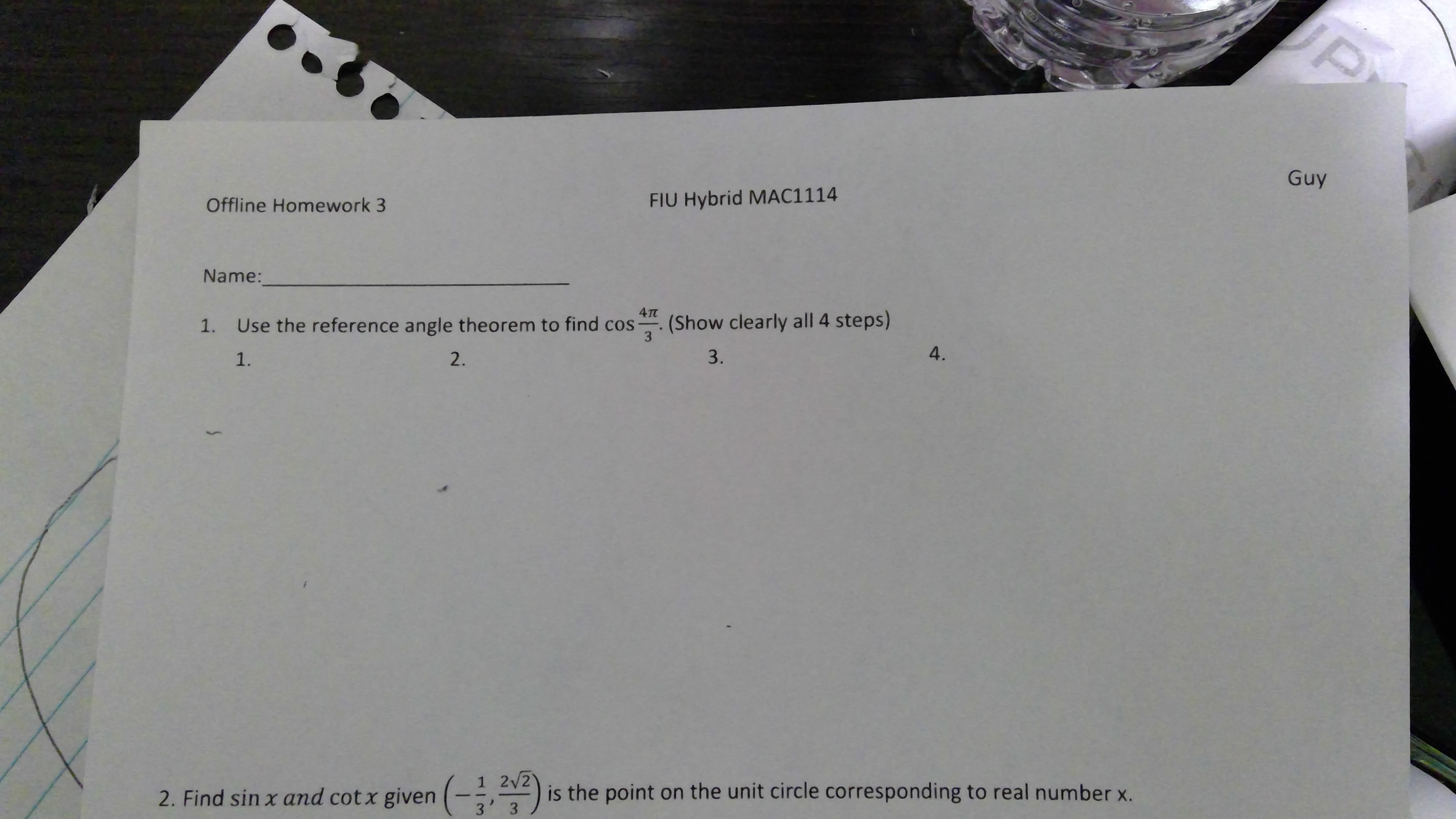# JPGuyFIU Hybrid MAC1114Offline Homework 3Name:(Show clearly all 4 steps)3Use the reference angle theorem to find cos-1.4.3.2.1.1 2/2is the point on the unit circle corresponding to real number x.32. Find sin x and cot x given (-, )

Question
1 views

How do you solve this and can you show me step by step?help_outlineImage TranscriptioncloseJP Guy FIU Hybrid MAC1114 Offline Homework 3 Name: (Show clearly all 4 steps) 3 Use the reference angle theorem to find cos- 1. 4. 3. 2. 1. 1 2/2 is the point on the unit circle corresponding to real number x. 3 2. Find sin x and cot x given (-, ) fullscreen
check_circle

Step 1

“Hey, since there are two questions posted, we will answer first question (1). If you want any specific question to be answered then please submit that question only or specify the question number in your message.”

Step 2

Reference angle Theorem:

The value of trigonometric function of a reference angle is equal to the value of trigonometric function of corresponding angle. Here the sign of trigonometric function depends on the quadrant where the angle lies.

Step 3

Here,

...

### Want to see the full answer?

See Solution

#### Want to see this answer and more?

Solutions are written by subject experts who are available 24/7. Questions are typically answered within 1 hour.*

See Solution
*Response times may vary by subject and question.
Tagged in

### Other